## Wednesday, August 5, 2015

### How much to tip at a restaurant? (Part 2 of 2)

How much to tip at a restaurant? (Part 2 of 2)

## The method

### Linear regression model

Now, we we’ll try to use the bill amount to help us improve our model. But first, let’s see if this variable has indeed some effect in the prediction of the tip.
Let’s start by plotting our data and then try to observe any possible relation.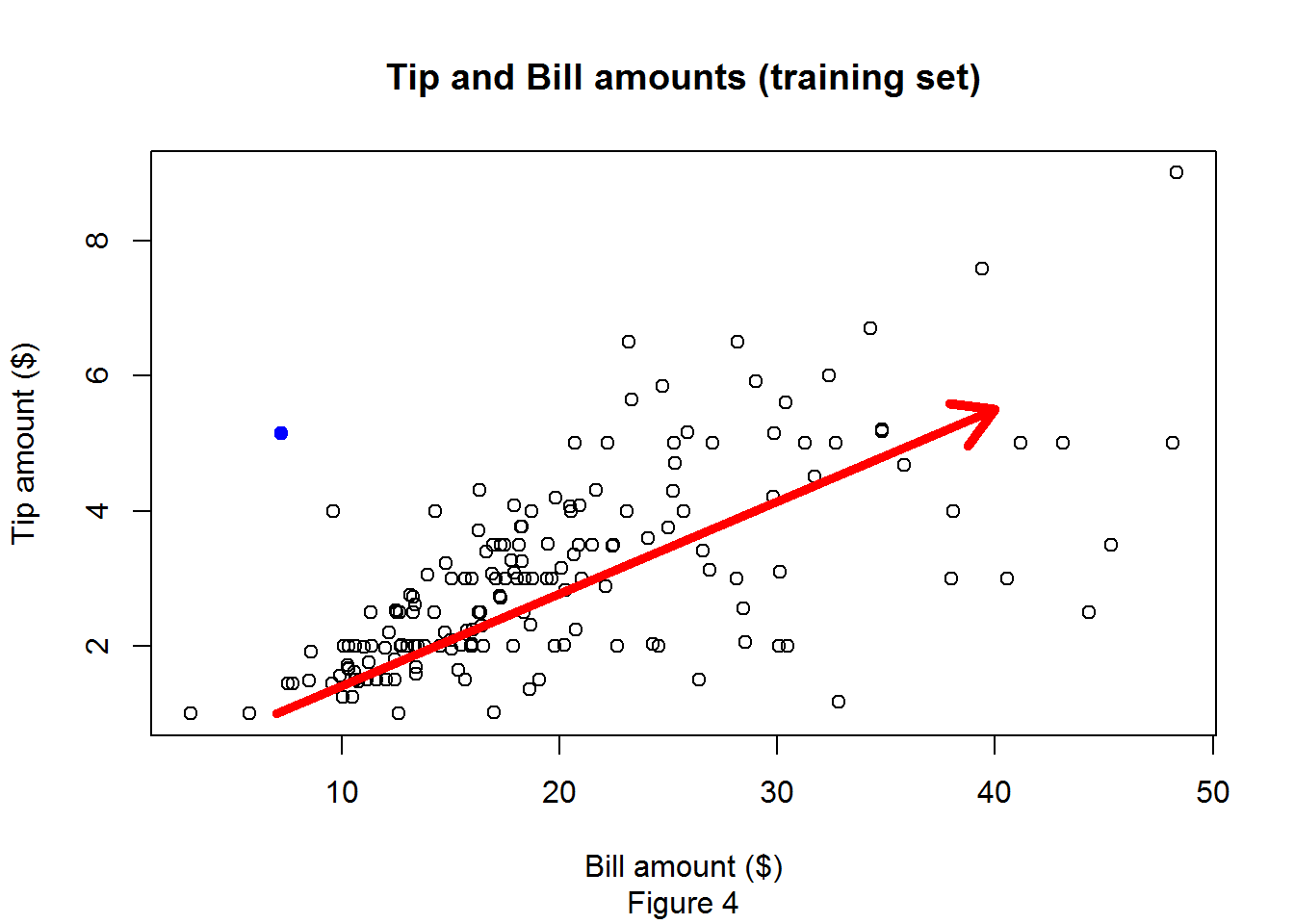We observed in Figure 4 the tip tendency to increase as the bill increased (red arrow). This is not a perfect linear relationship, but still is very good. Also the graphic shows some points that are clearly far away from the tendency (ex. left blue point) , these points are known as outliers and can influence the behavior of our model (for the moment we will ignore them).
Our scatter plot gave us a hint about a possible relationship between our two variables, but how to measure this relationship? this is the function of the “correlation coefficient”. This coefficient captures the strength of the relationship between two variables. The values of this measure can vary between -1 and 1, with the following meaning:
• (0). No relationship.
• (-1). Strong negative relationship. Increase in one variable is perfectly related with a decrease in the other variable.
• (+1). Strong positive relationship. Increase in one variable is perfectly related with an increase in the other variable.
In practice it’s almost impossible to find a perfect negative or positive correlation, instead we will find values between them. Also it is worth mentioning that correlation does not imply causation. A typical example of a false causality is the increase in drowning deaths as the sales in ice cream increases, despite that they are correlated that doesn’t mean that one causes the other, instead both can be caused by a third variable, in this case the summer, the high temperature in summer causes people to increase their consumption of icecream and also summer is a period of time when people engage more in outdoor activities like swimming.
Returning to our analysis, the correlation of our two variables is 0.64 which tell us that there is potential to use the bill_amount to predict the tip (the accepted strength of the correlation depends on the domain). We will not cover the details of how the correlation is calculated, but any statistical software can compute it in a single line a code (even excel).
Now that we have a hint about the relationship between tips and bills, we can try to improve our base model. The approach that we are going to use to build our model is linear regression.
Linear regression  is an approach that is used to model the relationship between one dependent variable and one or more independet variables. Usually this approach is used for prediction of the values of the depenent variable (in this case the tip amount) using the independent variables (bill amount). A good way to undestand linear regression with two variables (in a scenario like the one in this post) is by considering that linear regression tries to draw a line through the points minimizing the sum of squares errors (SSE), this is the distance between the point and the computed line.
In the previous post (Figure 3) we calculated the Sum of Squares Total (SST) for the average tip amount. This case is similar, but instead of using only the average of the tips we’ll also use the bill amount.
The next line of code shows how you can actually build and a linear regression model using the data analysis software R (https://www.r-project.org/).
model<-lm(tip~total_bill,data=train)
Now, let’s analize each part of this line of code.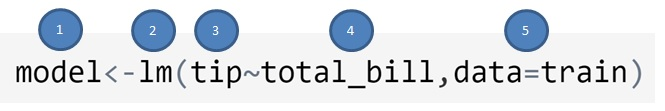1. A variable to save our model.
2. The funcition that acutally computes the linear regression model.
3. Our dependent variable.
4. Our independent variable.
5. The training set with our independent and dependent variables.
The output of linear regression can be seen as the blue line in Figure 5. This is the optimal straight line minimizing the SSE.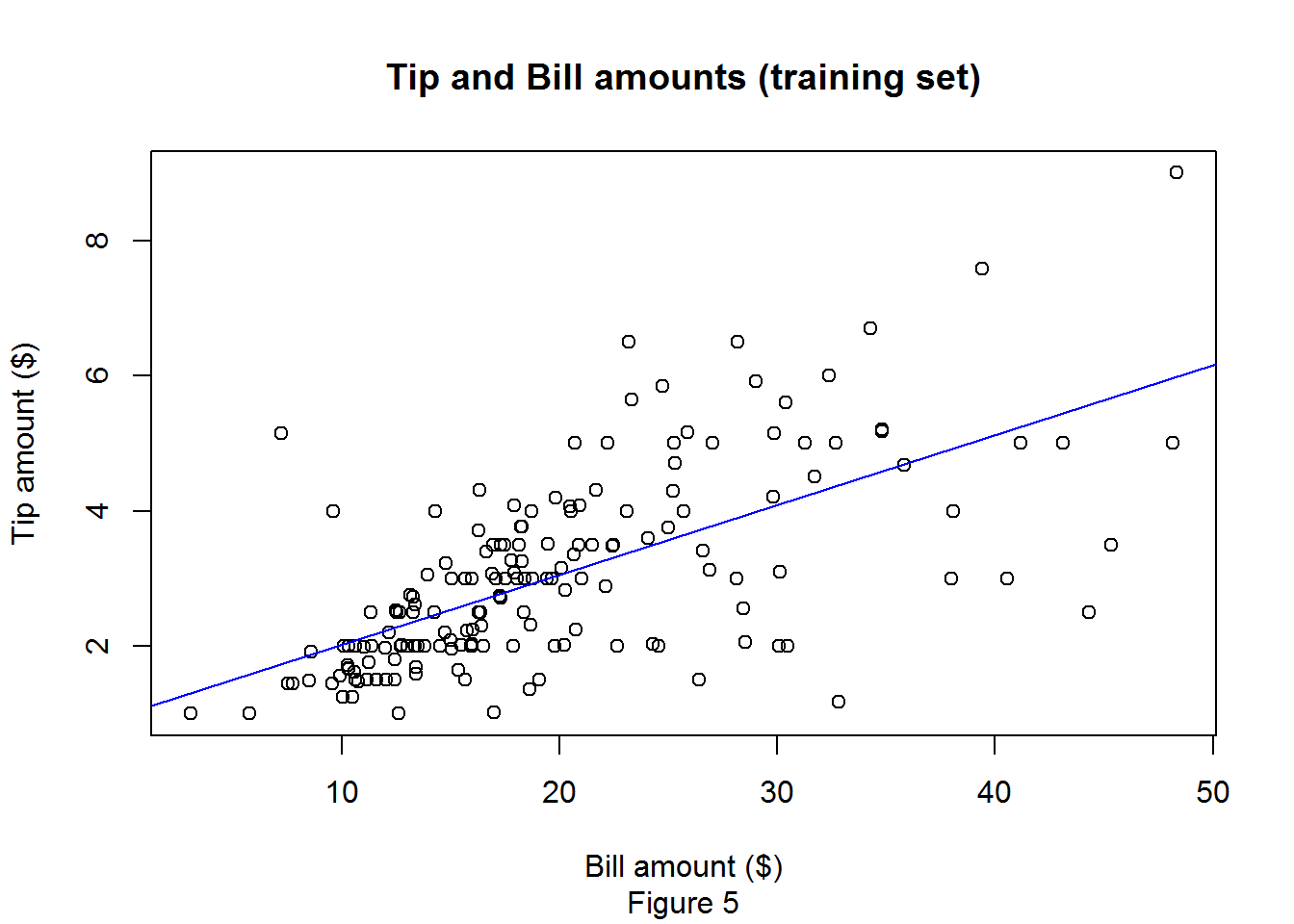## Results

Now that we have our model, it’s time to see how well it predicts values on the testing set, if you remember this is a set of data that we didn’t use for training, so our model had never seen this data. The following line of code can be used to get predictions on the test set.
predictions<-predict(model,newdata=test)
Again, let’s analize the line of code.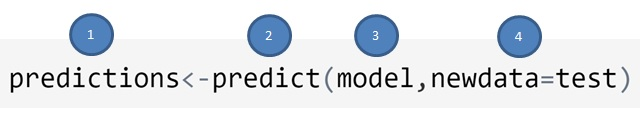1. A variable to save the predicted tips.
2. The function used to compute the predictions.
3. Our model, in this case our linear regression model.
4. The testing set with the independent variable.
Let’s see the behaviour of our model on the testing set. We ploted the same line as in Figure 5, but this time over the testing set.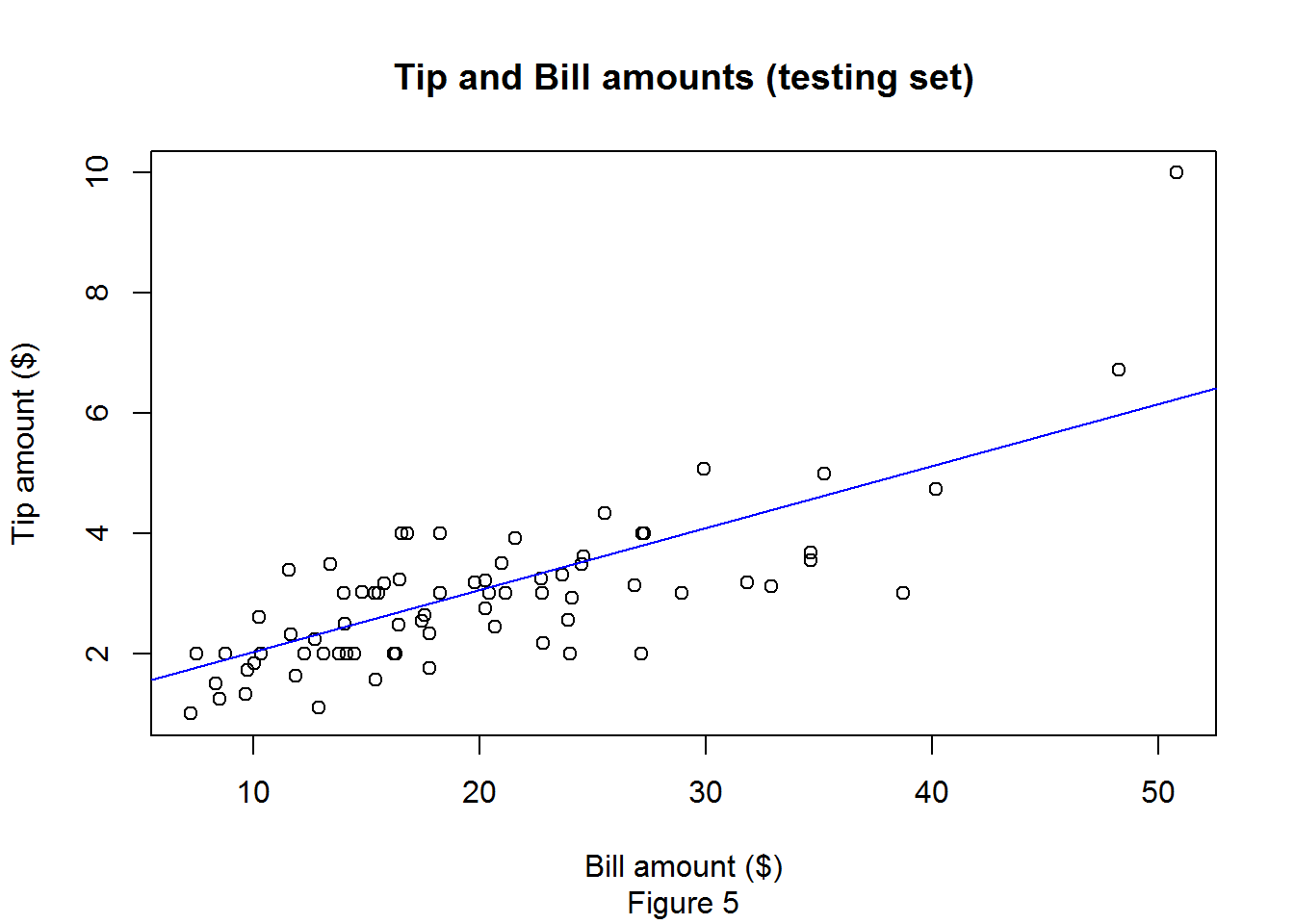Now we have our linear regression model to predict tips based on bills, everything represented as a straight line.
A way to measure how well our model behaved on the testing set is by computing the r2. This measure tell us how close the data is to the predicted line (Blue line in Figure 5), this mean the percentage of the dependent variable that is explained by the independent variable. The computation of r2 (Equation 1) on the testing set takes into account the SST of the base model $$r^2=1-(SSE/SST)$$, this means that we can use it to determine if there is an improvement over the based model. The value of r2 can vary between 0 and 1:
• (0). There is no linear relationship between our independent and dependent variable. The base model is the best fit. This means that we are better using only the mean of the tips.
• (1). All the points lie in a perfect straight line. The relation is perfectly linear.
We computed r2 on the testing set for our dataset with the formula $$r^2=1-(SSE/SST)$$ and obtained a value of 0.84, which actually is pretty good, so our model is doing a very good job and is better that the base model.
Finally we have our model, we know how well it fits the data and we have predicted values for our testing set, but let’s see how you can use it to predict your own tips. This is very easy using the regression formula: $$tip=\beta_{0}+\beta_{1}(bill)$$. If this is confusing to you try to analize each element of the formula:
• ($$tip$$). The value that we are trying to predict.
• ($$\beta_{0}$$). The intercept of the the regression line and the y axis.
• ($$\beta_{1}$$). The slope of the regression line
• ($$bill$$). The bill amount.
Then we need 3 values to compute the tip, the ($$bill$$) is your own bill amount, and $$\beta_{0}$$ and $$\beta_{1}$$ can be obtained in a single line in code in R:
## (Intercept)  total_bill
##   0.9926563   0.1032314
Then we have that tip=0.9926563+0.1032314(bill), so you need only to substitute bill by your own bill and you’ll have a tip prediction. For example let’s suppose that last week i invited you to dinner and the amount of the bill was $50, using our model we have that tip=0.9926563 + 0.1032314 x 50, tip=$5.12.
Despite that it took me 2 posts to explain simple linear regression, in practice it is really easy to use. In R you can build your regression model and compute the predicted values with two lines of code, but i believe that an important quality of a data analyst is to understand the model that he is using and not only to have the technical ability to do it without any theoretical understanding.

## Final thoughts

In this post we use simple linear regression, here simple means that we are using only one independent variable to predict our dependent variable, in practice we usually want to use more variables to help us build a better model, this is known as multiple linear regression. Besides $$r^2$$, a residual plot can reveal patterns that can indicate a biased result.
Finally, in some scenarios it is worth trying to eliminate outliers before building the model, these outliers can have a large impact and their incorportation or not in the model must be done with caution.
1. Montgomery, Douglas C., Elizabeth A. Peck, and G. Geoffrey Vining. Introduction to linear regression analysis. Vol. 821. John Wiley & Sons, 2012.